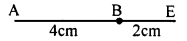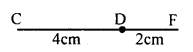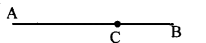# KSEEB Solutions for Class 8 Maths Chapter 3 Axioms, Postulates and Theorems Ex 3.1

In this chapter, we provide KSEEB SSLC Class 8 Maths Chapter 3 Axioms, Postulates and Theorems Ex 3.1 for English medium students, Which will very helpful for every student in their exams. Students can download the latest KSEEB SSLC Class 8 Maths Chapter 3 Axioms, Postulates and Theorems Ex 3.1 pdf, free KSEEB SSLC Class 8Maths Chapter 3 Axioms, Postulates and Theorems Ex 3.1 pdf download. Now you will get step by step solution to each question.

## Karnataka Board Class 8 Maths Chapter 3 Axioms, Postulates and Theorems Ex 3.1

Question 1.
What are undefined objects in Euclid’s geometry?
Certain objects in mathematics which cannot be defined using the terms an already know. Lines, points, plane, space are undefined objects in Euclidean geometry.

Question 2.
What is the difference between an axiom and a postulate?
Axiom :

1. Axioms are elementary statements, which are self-evident and which are accepted without questions.
2. Axioms are applicable to all branches of mathematics and science.

Postulate:

1. The statements which are particular to geometry and accepted without question are called geometrical postulates.
2. Postulates are applicable to geometry only.

Question 3.
Give an example of the following axioms from your experience.
a. If equals are added to equals, the wholes are equal.
b. The whole is greater than the part.
a. In the fig. AB = CD = 4cm BE = DF = 2 cmAB + BE = 4 + 2 = 6cm ……….(i)
CD + DF = 4 + 2 = 6cm ……….(ii)From (i) and (ii) AE = CF
AB = AC + CBCompare AB,AC and CB you find AB > AC and AB > CB.

Question 4.
What is the need of introducing axioms?
The Greek mathematicians faced great difficulty while developing geometry as a pure deductive science. They had to depend on certain primitive notions like points, line and planes and space. But this was not enough to deduce everything. They had to set up certain statements whose validity was accepted unquestionably. Thus there was a need of introducing axioms.

Question 5.
You have seen earlier that the set of all-natural numbers is closed under addition (closure property). Is this an axiom or something you can prove?
Yes, this can be considered as an axiom

All Chapter KSEEB Solutions For Class 8 maths

—————————————————————————–

All Subject KSEEB Solutions For Class 9

*************************************************

I think you got complete solutions for this chapter. If You have any queries regarding this chapter, please comment on the below section our subject teacher will answer you. We tried our best to give complete solutions so you got good marks in your exam.

If these solutions have helped you, you can also share kseebsolutionsfor.com to your friends.

Best of Luck!!